Select Page

# 12 Science CBSE Solutions for MCQ Maths Continuity and Differentiability in English

12 Science CBSE Solutions for MCQ Maths Continuity and Differentiability in English to enable students to get Solutions in a narrative video format for the specific question.

Expert Teacher provides 12 Science CBSE Solutions for MCQ Maths Continuity and Differentiability through Video Solutions in English language. This video solution will be useful for students to understand how to write an answer in exam in order to score more marks. This teacher uses a narrative style for a question from Continuity and Differentiability not only to explain the proper method of answering question, but deriving right answer too.

Please find the question below and view the Solution in a narrative video format.

Question:

Solution Video in English:

You can select video Solutions from other languages also. Please check Solutions in ( Hindi )

## Similar Questions from CBSE, 12th Science, Maths, Continuity and Differentiability

Question 1 : Findfor the function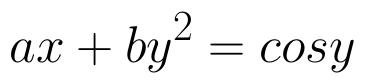. (View Answer Video)

Question 2 : If x and y are connected parametrically by the equation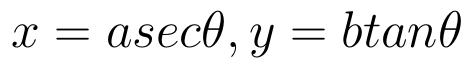, without eliminating the parameter, find. (View Answer Video)

Question 3 : If x and y are connected parametrically by the equation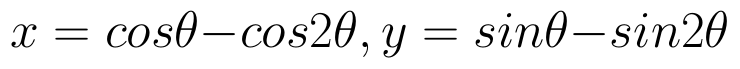, without eliminating the parameter, find. (View Answer Video)

Question 4 : Differentiate the function w.r.t.x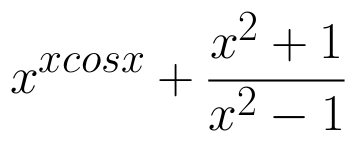. (View Answer Video)

Question 5 : Differentiate w.r.t.x the function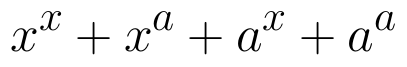, for some fixed a > 0 and x > 0. (View Answer Video)

### Three Dimensional Geometry

Question 1 : Find the cartesian equation of the line which passes through the point (-2, 4, -5) and is parallel to the line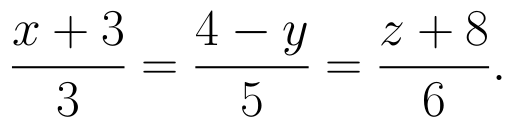(View Answer Video)

Question 2 : Find the angle between the planes 7x + 2y + 6z = 15 and 3x - y + 10z = 17. (View Answer Video)

Question 3 : If a lines makes angle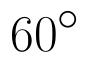and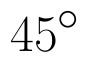with the positive directions of x-axis and z-axis respectively, then find the angle that it makes with the y-axis. (View Answer Video)

Question 4 : Find the equation of the plane passing through the point (-1, 3, 2) and perpendicular to each of the planes x + 2y + 3z = 5 and 3x + 3y + z = 0. (View Answer Video)

Question 5 : A line passes through the point with position vector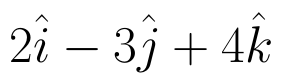and makes angles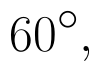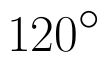andwith x, y and z-axis respectively. Find the equation of the line in the Cartesian form. (View Answer Video)

### Differential Equations

Question 1 : If m and n are the order and degree, respectively of the differential equation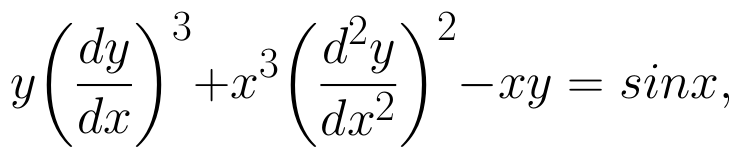then write value of m+n. (View Answer Video)

Question 2 : Solve the differential equation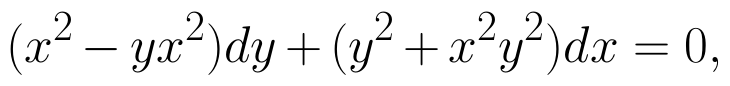given that y = 1 when x = 1. (View Answer Video)

Question 3 : If x cos(a + y) = cos y, then prove that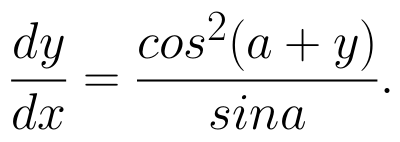Hence show that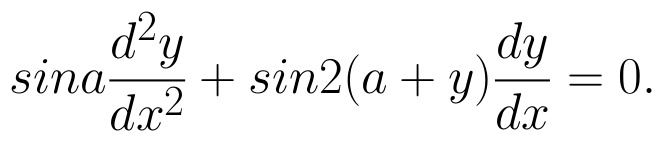(View Answer Video)

Question 4 : Find the particular solution of the differential equation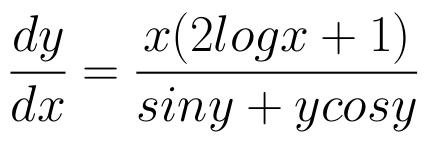given that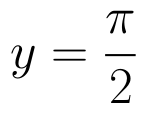where x = 1. (View Answer Video)

Question 5 : Solve the differential equation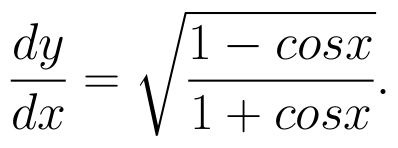(View Answer Video)

### Vector Algebra

Question 1 : Find the value of x and y so that the vectors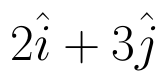and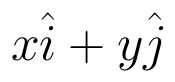are equal. (View Answer Video)

Question 2 : Find the sum of the vectors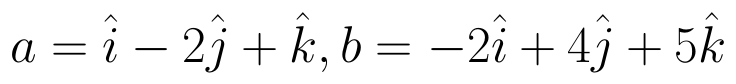and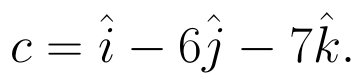(View Answer Video)

Question 3 : Find the angle between two vectors a and b with magnitudes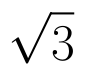and 2 respectively, having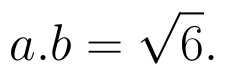(View Answer Video)

Question 4 : L and M are two points with position vectors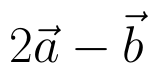and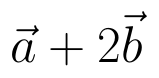respectively. Write the position vectors of a point N which divides the line segment LM in the ratio 2:1 externally.   (View Answer Video)

Question 5 : Find the magnitude of two vectors a and b having the same magnitude and such that the angle between them isand their scalar product is 1/2. (View Answer Video)